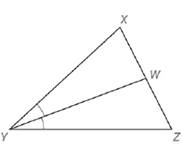Chapter 5.6, Problem 35EElementary Geometry For College St...

7th Edition
Alexander + 2 others
ISBN: 9781337614085

Solutions

Chapter
SectionElementary Geometry For College St...

7th Edition
Alexander + 2 others
ISBN: 9781337614085
Textbook Problem

Use Theorem 5.6.3 to complete the proof of this theorem: “If the bisector of an angle of a triangle also bisects the opposite side, then the triangle is an isosceles triangle.” Given: Δ X Y Z ; Y W → bisects ∠ X Y Z ; W X ¯ ≅ W Z ¯Prove: Δ X Y Z is isosceles (HINT: Use a proportion to show that Y X = Y Z . )

To determine

To prove:

ΔXYZ is isosceles.

Explanation

Given:

We need to prove, “If the bisector of an angle of a triangle also bisects the opposite side, then the triangle is an isosceles triangle”.

In a ΔXYZ, YW bisects XYZ; WX¯WZ¯.

Theorem used:

Angle bisector theorem:

If a ray bisects one angle of a triangle, then it divides the opposite side into segments whose lengths are proportional to the lengths of the two sides that form the bisected angle.

Proof:

Consider a ΔXYZ.

Given that YW bisects XYZ.

By angle bisector theorem, we get

WXWZ=YXYZ … (1)

Given that WXx

Still sussing out bartleby?

Check out a sample textbook solution.

See a sample solution

The Solution to Your Study Problems

Bartleby provides explanations to thousands of textbook problems written by our experts, many with advanced degrees!

Get Started

Prove the statement using the precise definition of a limit. limx2(145x)=4

Single Variable Calculus: Early Transcendentals, Volume I

Change 64 ft/s to mi/h.

Elementary Technical Mathematics

A population of N = 7 scores has a mean of = 13. What is the value of X for this population?

Essentials of Statistics for The Behavioral Sciences (MindTap Course List)

In Exercises 6372, evaluate the expression. 63. |6 + 2|

Applied Calculus for the Managerial, Life, and Social Sciences: A Brief Approach

True or False: n=1(1)nn+14 is a convergent series.

Study Guide for Stewart's Single Variable Calculus: Early Transcendentals, 8th